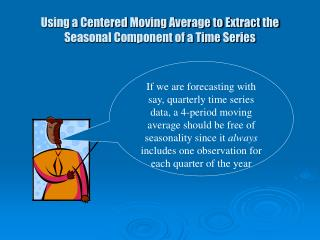DownloadDownload PresentationUsing a Centered Moving Average to Extract the Seasonal Component of a Time Series

# Using a Centered Moving Average to Extract the Seasonal Component of a Time Series

Télécharger la présentation## Using a Centered Moving Average to Extract the Seasonal Component of a Time Series

- - - - - - - - - - - - - - - - - - - - - - - - - - - E N D - - - - - - - - - - - - - - - - - - - - - - - - - - -
##### Presentation Transcript

1. Using a Centered Moving Average to Extract the Seasonal Component of a Time Series If we are forecasting with say, quarterly time series data, a 4-period moving average should be free of seasonality since it always includes one observation for each quarter of the year

2. Suppose we have a quarterly time series X1, X2, X3, . . . , Xn The first value that can be calculated for this series by a 4-period MA process would use observations X1, X2, X3, and X4. Notice that our first 4-period average has a center between quarter 2 and quarter 3. Hence we will designate it X*2.5. Thus we have: The next value is:

3. For the series X1, X2, X3, . . . , Xn, the formula is1 : (1) This algorithm gives us a series that is free of seasonality. Alas, the location of the values of this series do not correspond to the original series.

4. We can correct this problem with acentered moving average If we average adjacent pairs of X*t’s, we obtain a series that is free of seasonality and is aligned correctly with our original series

5. To get a 4-period moving average that is centered at quarter 3 (designated by X3**), take the average of X2* and X3*: The general formula is: (2)

6. Combining equations (2) and (3), the series Xt** can be expressed by a weighted moving average: The seasonal index (St) can be computed by dividing Xt by Xt**. That is:

7. Example: Quarterly product sales • Notice we lose 2 data points at the beginning of the series, and 2 at the end. • For monthly data, we would lose a total of 12 data points.